﻿﻿ Permeanceexamples｜products｜Murata Software Co., Ltd.# Example26Permeance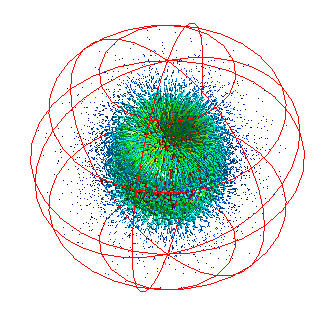General

• The permeance of a magnet is calculated.

• Unless specified in the list below, the default conditions will be applied.

### Analysis Conditions

 Item Settings Solvers Magnetic Field Analysis [Gauss] Analysis Space 3D Analysis Type Static analysis Model unit mm Options N/A

### Model

The permeance of a spherical magnet is calculated.

The magnetizing direction of the magnets is +Z by default.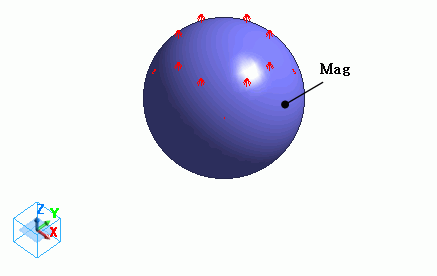### Body Attributes and Materials

 Body Number/Type Body Attribute Name Material Name 1/Solid mag mag

* Available from the Material DB

The material properties are set up as follows:

 Material Name Tab Properties mag Permeability Material Type: Permanent Magnet Magnet Magnetization Strength: 1.0

### Boundary Conditions

Open boundary is set for the outer boundary condition.

 Boundary Condition Name/Topology Tab Boundary Condition Type Settings Outer Boundary Condition Electric Open boundary

### Results

Permeance coefficient is given by

Pc = B/u0H

where B is the magnetic flux density [T], H is the magnetic field [A/m] and u0 is the permeability of vacuum, 4πx10-7.

Pc of a particular point in magnet can be calculated from B and H at that point..

• The distribution of permeance can be viewed with contour diagram.
See [User-Defined Field] for the detail.

It is constant in case of spheroids.

B and H at a particular point can be obtained as follows.

##### Calculation of Permeance Coefficient

Select [Magnetic flux density] at Field in the Display group on the [Results] tab.Click [Results at Specified Coordinates]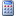.Enter the coordinates of the point. For example, enter (0, 0, 0).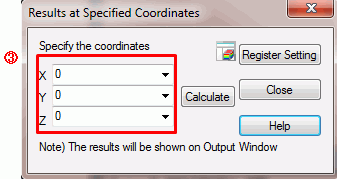Click the Calculate button. [Magnetic flux density] will be calculated and displayed on the screen.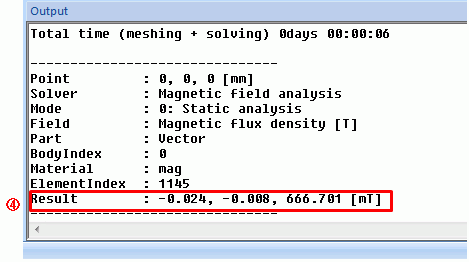Then, change the Field from [Magnetic flux density] to [Magnetic field].Calculate [Magnetic field] in the same manner.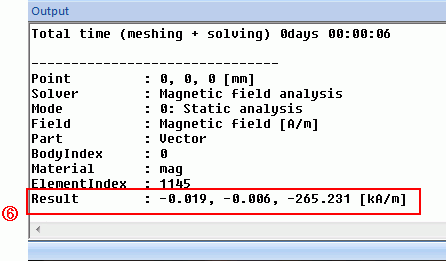The permeance coefficient can be calculated from their z components.

Pc = 6.666876e-001 / 2.652416e+005 / u0 = 2.000188

It is very close to the theoretical value of 2.

﻿ ﻿